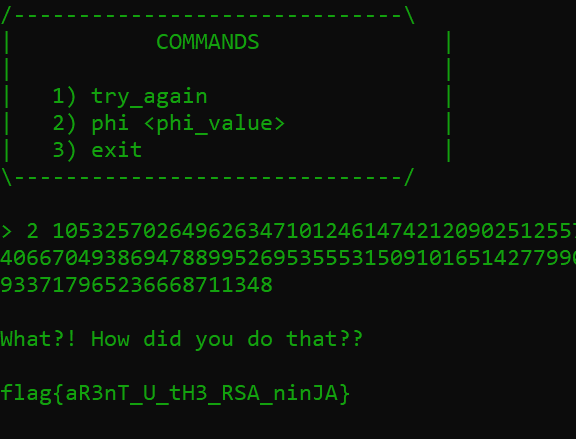# fr334aks

## A blog for the fr334aks CTF Team.

14 September 2022

# Writeup For 'Phi Too Much in Common' From CSAW CTF 2022

by 0xManticore

Hey hackers, I was exploring some of the cryptography challenges in CSAW CTF, I managed to solve this one and loved to share my approach.

### Content Tree:

Challenge Name: Phi Too Much in Common The challenge is divided into two small challenges, we can check each of them individually

## First Part (`Common Modulus Attack`):

After connecting to the server given, we receive values for `n, e, c`.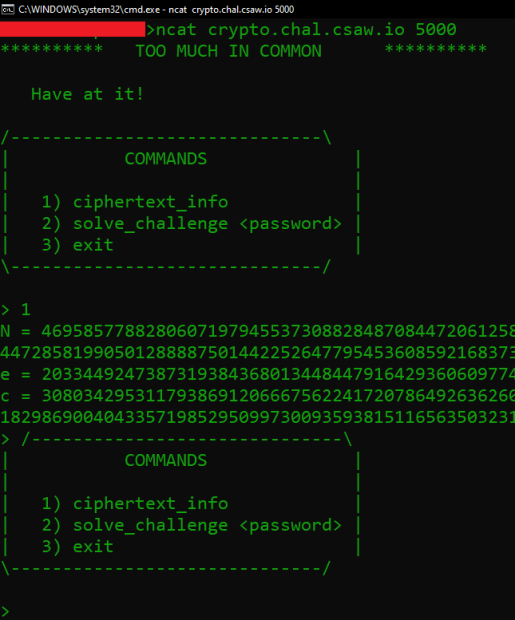As we can see, the value of `n` is not small enough to be factored (309 digits), but the value of e is quite big, this suggests that d value might be small, and the cryptographic system is vulnerable to Wiener’s attack.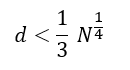However, I failed. This approach couldn’t retrieve the private key (`d value`).

Then I noticed that I can request another set of values from the server, with the word “Common” in the challenge name, I thought about two possibilities, first is that I will find two `n` values with a common prime, then from `p=gcd(n1,n2)` I can continue to calculate the rest. The other possibility is that the same n value will appear again but with different e value. So, we have one `n` value, two `e` values, and two encrypted messages, and this will be clearly a common modulus attack.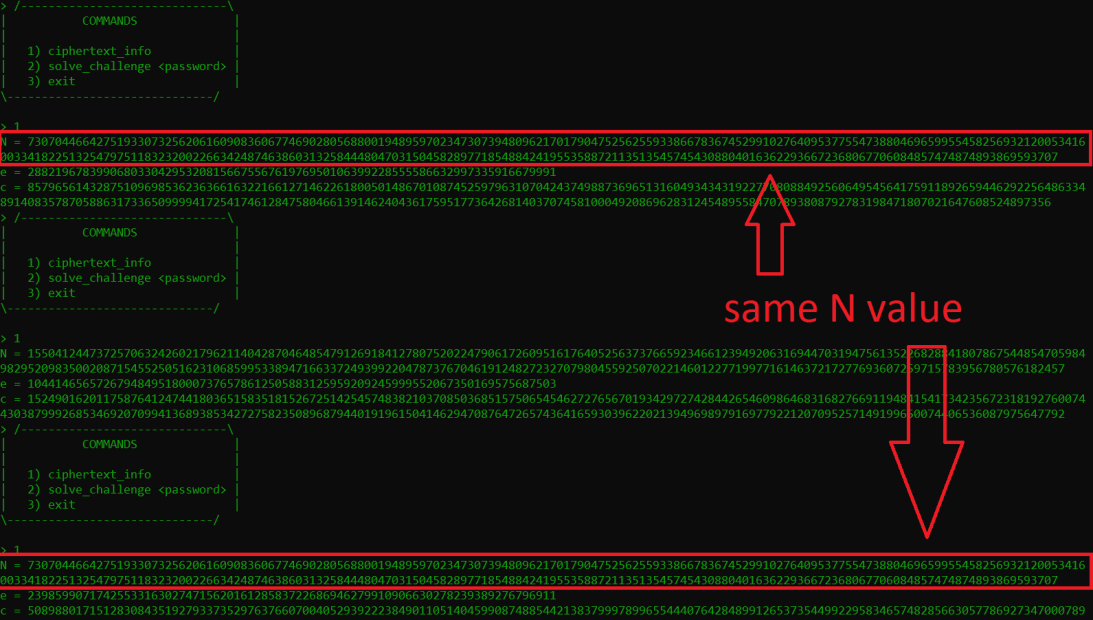And exactly as I thought, some of the n values kept appearing repeatedly. so, we go with the second option.

How common modulus attack works?

In an RSA cryptographic system where we have the same message `m` being encrypted twice using two different public exponents `e1` and `e2`. Knowing the values of `e1` and `e2` we can calculate `x` and `y` to satisfy the formula below using extended euclidean algorithm: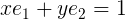Then substituting the values into this formula will reveal the original message: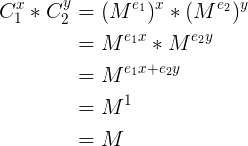To make things simpler, I used a python script inspired from APogiatzis with minor edits to work on python3 instead of python2, and it gives us the message:

``````from math import gcd

e1 = 28821967839906803304295320815667556761976950106399228555586632997335916679991
e2 = 23985990717425533163027471562016128583722686946279910906630278239389276796911
n = 73070446642751933073256206160908360677469028056880019489597023473073948096217017904752562559338667836745299102764095377554738804696599554582569321200534160033418225132547975118323200226634248746386031325844480470315045828977185488424195535887211351354574543088040163622936672368067706084857474874893869593707
ct1 = 8579656143287510969853623636616322166127146226180050148670108745259796310704243749887369651316049343431922770808849256064954564175911892659446292256486334891408357870588631733650999941725417461284758046613914624043617595177364268140370745810004920869628312454895584707893808792783198471807021647608524897356
ct2 = 50898801715128308435192793373529763766070040529392223849011051404599087488544213837999789965544407642848991265373544992295834657482856630577869273470007898274557803721742092021774876015792361046909230859759257227261715728185941660477870236733018196750282253564664641446613442945923720188055146971633823979354

def egcd(a, b):
if a == 0:
return (b, 0, 1)
else:
g, y, x = egcd(b % a, a)
return (g, x - (b // a) * y, y)

def modinv(a, m):
g, x, y = egcd(a, m)
return x % m

def attack(c1, c2, e1, e2, N):
s1 = modinv(e1,e2)
s2 = (gcd(e1,e2) - e1 * s1) // e2
temp = modinv(c2, N)
m1 = pow(c1,s1,N)
m2 = pow(temp,int(-s2),N)
return (m1 * m2) % N

def main():

message = attack(ct1, ct2, e1, e2, n)
print(f"Plain Text: {bytes.fromhex(hex(message)[2:]).decode()}")

main()
``````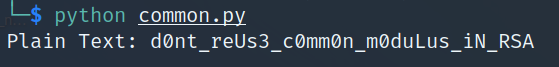submitting this back to the server gives us the second part of the challenging, which was quite new to me.

## Second Part: (`Finding Phi`):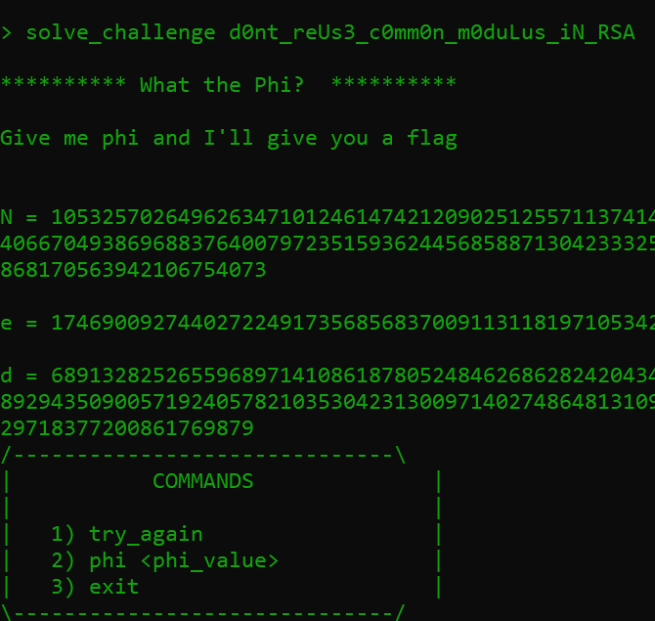in this part we are given `d` as well, but we need to compute `phi`, I have never encountered such scenario before, and my poor mathematical skills couldn’t find out how to get back phi value, I tried requesting multiple sets of values to see if that can help me crack the system, but no luck. After super-intensive search, I found this NIST Special Publication 800-56B R1 Recommendation for Pair-Wise Key Establishment Schemes Using Integer Factorization Cryptography in page 118 (page 129 in the pdf file), there is an algorithm that can help us achieve our goal (Calculate p and q from n,e,d), therefore calculating phi easily.

I wrote the same algorithm in a python script and used it to get phi value.

``````from math import gcd
from random import random

n = 105325702649626347101246147421209025125571137414407736295169432944228288162866542981537092562793854976446222920035896391970147115630011328339540667049386968837640079723515936244568588713042333254095564573657558219275895849493816962949027883577145703324976690982868239667669147634726100294868170563942106754073
e = 17469009274402722491735685683700911311819710534279648186423660629869466637691
d = 68913282526559689714108618780524846268628242043484228113366817148684807297089791376717618624780844488729160126266216119079245542599144234067668929435090057192405782103530423130097140274864813109715612092404358582453935553929088212492080562863512450851516765225879529063476431966000480706129718377200861769879

def find_phi(n,e,d):
k = e*d - 1
if k%2 == 1:
raise ValueError("### CANNOT BE HACKED ###")
r = k
t=0
while r % 2 == 0:
r = r//2
t+=1
for i in range(1,100):
g=int(random()*int(n-1))
y = pow(g,r,n)
if y == 1 or y == n-1:
continue
for j in range(1,11):
x = pow(y,2,n)
if x == 1:
break
if x == n-1:
continue
y=x
x=pow(y,2,n)
if x == 1:
break
p=gcd(y-1, n)
q = n//p
phi = (p-1) * (q-1)

return p,q,phi

def main():
p,q,phi = find_phi(n,e,d)
if n == p * q:
print("### SUCCESS ###")
print(f"p = {p}")
print(f"q = {q}")
print(f"phi = {phi}")
else:
raise ValueError("### FAILED ###")

main()
``````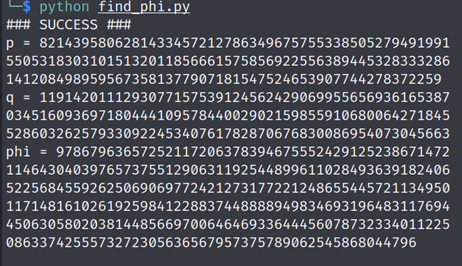after passing phi value to the server, I receive the flag.